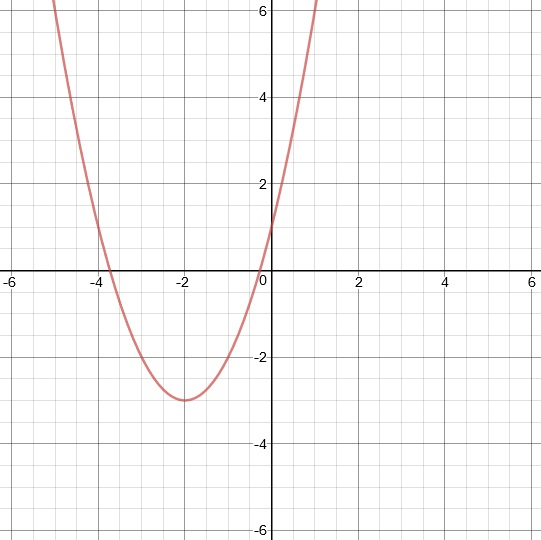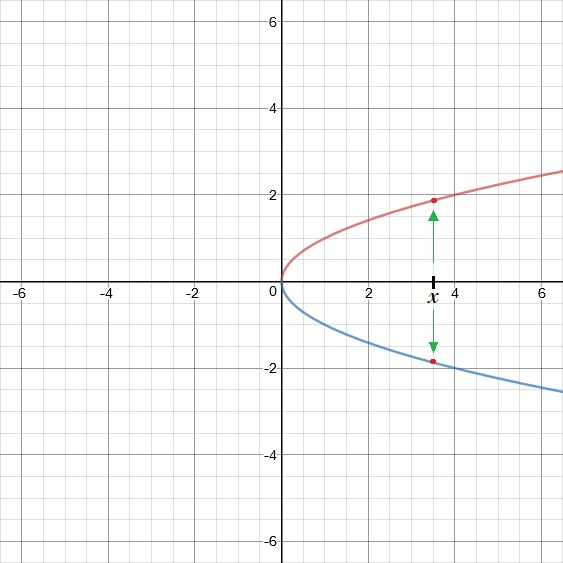Operations and Evaluations of Functions

Operations and Evaluations of Functions

function is a relation which states that for every possible x there is one and only one output for y

A relation is a function if it passes a vertical line test -- meaning that no vertical lines intersect the graph at more than one point.function                                                        not a function

The notation f(x) means that we have a function named f and the variable in that function is x. (It does NOT mean "f times x.")

The domain of the function describes values of x that can be put into the function. The range of the function describes the values of the output.

Evaluating a Function

Evaluating a function means to substitute a variable with its given number or expression.

Example

Evaluate f(x) = 2x + 4 for x = 5

This means to substitute 5 for x and simplify. It is recommended that the value being substituted be placed inside parentheses. (This is just to make things easier to look at.)

f(5) = 2(5) + 4 = 14

To add functions, add their outputs; to subtract functions, subtract their outputs.

Example

Given f(x) = x2 and g(x) = 4x + 1

f(x) + g(x) = x2 + 4x + 1

(f + g)(x) = x2 + 4x + 1

f(x) − g(x) = x2 − (4x + 1) = x2 − 4x − 1   (remember the distributive property)

(fg)(x) = x2 − 4x − 1

Multiplying a Function by a Constant

To multiply a function by a constant, multiply the output by that constant.

Example

Given f(x) = 4x − 1, find 2f(x).

f(x) = 4x − 1

2f(x) = 2(4x − 1) = 8x − 2

Multiplying a Function by a Function

To multiply a function by another function, multiply the outputs.

Example

Given f(x) = 2x and g(x) = x + 1, find f(x)g(x

f(x)g(x) = (2x) × (x + 1) = 2x(x + 1)

f(x)g(x) = 2x2 + 2x